Select Page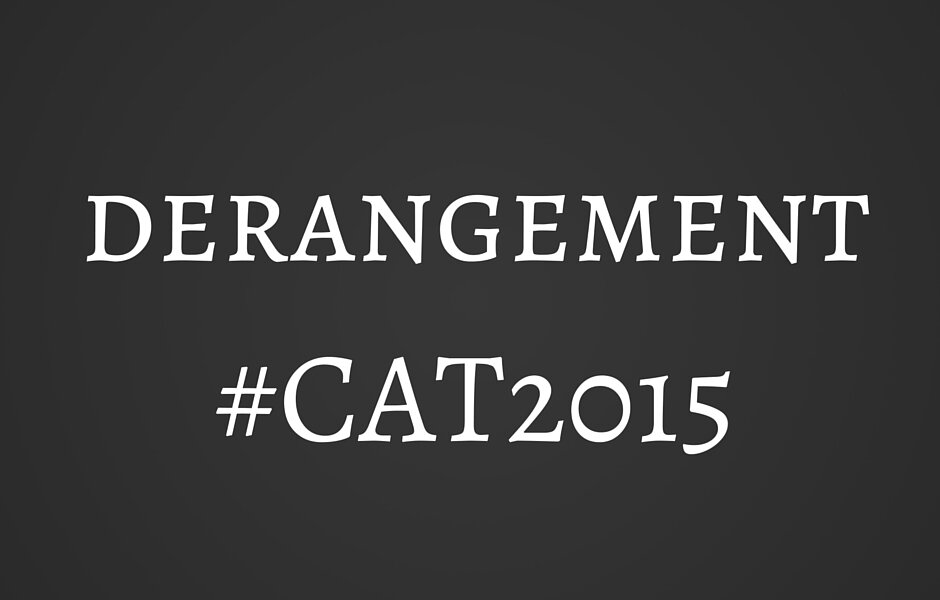Derangement is a concept in which, the questions essentially consist of de-arranging items i.e. if there is a particular relation between two objects, it has to be avoided while forming the arrangements. The questions are easy to pick and require only a couple of lines of calculation even in the worst cases.

Types of questions

1) Direct derangement question without any other condition

2) Conditional derangement

While the first one is strictly formula based, the second one requires a little bit of visualization and logic.

Direct derangement

Let’s understand this with the help of an example. Suppose there are 4 letters A, B, C and D which are yellow, red, green and blue in colour respectively. Also, there are 4 envelopes 1, 2, 3 and 4 which are again yellow, red, green and blue in colour respectively. So, the following arrangements are possible for (A, B, C, D):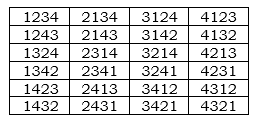Now, we have to find out all the cases such that A does not correspond to 1, B does not correspond to 2, C does not correspond to 3 and D does not correspond to 4. Remember that not even a single letter should coincide with the same coloured envelope. You get the following cases: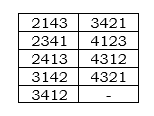So, 9 cases in total. You can find this number using the derangement formula: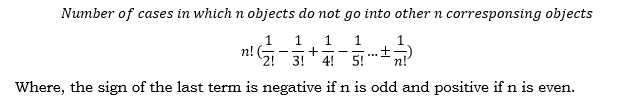So, in our case,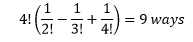You can get explicit questions from this area in various forms. Just remember that there have to be n objects which correspond to exactly n other objects and there should be a condition that there should not be even a single pair that is matched. Here are a few examples to try out:

1. Six couples go to a wedding function. There is a dance sequence wherein, not even a single husband should be with his wife. In how many ways can the arrangements be formed?
2. There are four students Arun, Barun, Kiranmala and Ghosh. They like to have idli, vada, dosa and upma respectively. In how many ways is it possible that none of them get what they like? Assume that these are the only four items available and in infinite supply.

Conditional derangement

In this case, the derangement will be masked by another condition. The number of objects marked to the wrong corresponding object will be lesser than the total number of pairs that can be formed. While it might sound a bit tricky, the procedure to solve these questions is a logical extension of the above type. Consider an example:

There are five letters marked 1, 2, 3, 4 and 5 and there are five envelopes 1, 2, 3, 4, 5. In how many ways can the letters be put into envelopes such that exactly one letter goes into the correct envelope?

The last part is where the trap lies. But the solution is pretty easy. You can fix the first letter into the first envelope and calculate for the remaining pairs. You can see that the remaining pairs have to be derangements for 4 items. So, the answer will simply be D(4) = 9 ways. Also, remember the fact that the fixed letter could be either of 1, 2, 3, 4 or 5 which can be done in 5c1 ways and so, we have to multiply the resultant by 5. So, 9*5=45 will be the answer.

Try out a similar question:

There are five letters marked 1, 2, 3, 4 and 5 and there are five envelopes 1, 2, 3, 4, 5. In how many ways can the letters be put into envelopes such that exactly two letters go into the correct envelopes?

Points to remember

1. Whenever (n-1) objects are in their right positions, the last object also has to be in the right position. So, if the question is:

There are five letters marked 1, 2, 3, 4 and 5 and there are five envelopes 1, 2, 3, 4, 5. In how many ways can the letters be put into envelopes such that exactly four letters go into the correct envelopes?

The answer would be 1 in this case. As, the four letters have been put into their corresponding envelopes, the fifth letter has to go to the right envelope as well.

1. However, the converse is not always true. Even if neither of the (n-1) objects have been placed at their designated positions, the remaining object could be placed at its correct position.
2. Remember the derangement values for at least the first 6 numbers: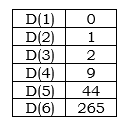Hope that clears the derangement concepts by a bit. Happy solving!

error: Content is protected !!